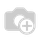# How filter x2many?

Is it possible filter list of x2many records in form view?

For example:

1)Show only first 5 lines.

2)Show only records, that have fields according to some domain.##### 3 응답

Regarding the change of the limit of one2many fields please refer to question https://accounts.openerp.com/forum/Help-1/question/6627/

Regarding the filter I had the same requirement for one of our modules and I have solved it with the following steps. Assume that you have the following one2many field which you want to filter:

``````'line_ids': fields.one2many('your.model', 'script_id', 'Lines'),
``````

``````'ir_filter_id': fields.many2one('ir.filters', 'Additional Filter', domain=[('model_id', '=', 'your.model')]),
``````

``````def _get_lines(self, cr, uid, ids, fields, args, context=None):
line_obj = self.pool.get('your.model')
res = {}
for script in self.browse(cr, uid, ids):
args = [('script_id', '=', script.id)]
if script.ir_filter_id:
args += eval(script.ir_filter_id.domain)
line_ids = line_obj.search(cr, uid, args)
res[script.id] = line_ids
return res
def _set_lines(self, cr, uid, id, name, value, inv_arg, context):
line_obj = self.pool.get('your.model')
for line in value:
if line == 1: # one2many Update
line_id = line
line_obj.write(cr, uid, [line_id], line)
return True
'view_line_ids': fields.function(_get_lines, fnct_inv=_set_lines, string='Lines', relation="your.model", method=True, type="one2many"),
``````

So you can filter your one2many field with any domain. You only have to be aware of the following points:

- The filter is stored in the DB and all users have the same filter - You have to save the form view so that the current filter becomes active

2 의견Big thanks for posting this, it worked nicely after adding also checks for line being 0 or 2 (create or delete). I think there shouldn't have to be this much magic involved when wanting to filter a basic o2m field, though.

Thanks to Andreas Brueckl for idea with second functional 'view' field, example first case may be (filter first 5):

``````def _get_lines(self, cr, uid, ids, name, arg, context=None):
line_obj = self.pool.get('your.model')
res = {}
number = 5
for script in self.browse(cr, uid, ids, context=context):
line_ids = line_obj.search(cr, uid,[('script_id', '=', script.id)], limit=number)
res[script.id] = line_ids
return res
'view_line_ids': fields.function(_get_lines, relation="your.model", method=True, type="one2many"),
``````Thanks to Andreas for this detailed answer, the fnct_inv definition saved me some time. I would suggest for others that might need it, you can add:

``````elif line == 0: # one2many create
self.create(cr, uid, line)
``````

to the _set_lines function if you want to create new record.